﻿
Reach Us+44-7482-875032
Method for Solving Polynomial Equations

# Journal of Applied & Computational MathematicsOpen Access

All submissions of the EM system will be redirected to Online Manuscript Submission System. Authors are requested to submit articles directly to Online Manuscript Submission System of respective journal.

# Method for Solving Polynomial Equations

Nahon YJ*
Department of Mathematics, Technion-Israel Institute of Technology, Ashdod, Israel
*Corresponding Author: Nahon YJ, Department of Mathematics, Technion- Israel Institute Of Technology, Ashdod, Israel, Tel: 00972-52-742-1339, Email: [email protected]

Received Date: Aug 21, 2017 / Accepted Date: Aug 04, 2018 / Published Date: Aug 07, 2018

Keywords: Polynomial Equations; Coefficients; Series; Recurrent Relationships; Finite number

#### Introduction

Nahon theorem

If a n0 th polynomial equation in powers of X:

X (n0)=A(n0-1)*X(n0-1)+A(n0-2)*X (n0-2)+A(n0-3)*X (n0- 3)+…..A(1)*X+A(0) with the condition in the coefficients 

A(n0-1)*A(0)≠0

has a solution X in the set of real numbers R then if we define a series E(n) with E(n0)=A(n0-1) and with the recurrent formula :

E(n+1)=A(n0-1)*E(n)+A(n0-2)*E(n-1)+A(n0-3)*E(n-2)+……. A(1)*E(n-n0+2)+A(0)*E(n-n0+1) for (n ≥ n0). Then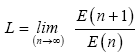is a solution of the n0 th polynomial equation . So X=L.

Condition of convergence : for i=1,2,…..(n-1)

A(i)≠0→[A(n0-1)*A(i)+A(i-1)]≠0 .

Proof of the Nahon theorem

X(n0)=A(n0-1)*X(n0-1)+A(n0-2)*X(n0-2)+A(n0-3)*X (n0-3)+….A(1)*X+A(0)

X(n0+1)=X(n0)*X

Multiplication of the two sides by X leads to :

X(n0+1)=A(n0-1)*X(n0)+A(n0-2)*X(n0-1)+A(n0-3)*X(n0-2)+… A(1)*X2+A(0)*X

Substituting X(n0)

by its expression in the second expression for X(n0+1) leads to :

X(n0+1)=A(n0-1)×[A(n0-1)*X (n0-1)+A(n0-2)*X (n0-2)+A(n0-3)*X(n0-3)+…

A(1)*X+A(0)]+A(n0-2)*X (n0-1)+A(n0-3)*X (n0-2)+….

A(1)*X2+A(0)*X=(Grouping together the coefficients of the same powers)

X(n0+1)=X(n0-1)× [A(n0-1)2+A(n0-2)]+X(n0-2)× [A(n0-1)*A(n0- 2)+A(n0-3)]+ X( n0-3) × [A( n 0 - 1 ) *A( n 0 - 3 ) +A( n0-4)]+…X × [A( n 0 - 1)*A(1)+A(0)]+A(n0-1)*A(0)

So in this manner by multiplication by X and then substitution of X(n0) by its expression we can express all the subsequent powers 

X(n0+2), X(n0+3), X(n0+4),……, X(n0+k)

in terms of a basis composed of the powers [X(n0-1), X(n0-2), X(n0-3),….X, X(0)]

So let’s denote the coefficients of each power contained in this basis by series E(n) ,

E(2(n)), E(3(n)),……..E(n0-1(n)),E(n0(n)) (For n ≥ n0)

So we can write

X(n0)=E(n0)*X(n0-1)+E(2(n0))*X(n0-2)+E(3(n0))*X (n0-3)+…E(n0-1(n0))*X+E(n0(n0))

Similarly we can write:

X(n0+1)=E(n0+1)*X (n0-1)+E(2(n0+1))*X(n0-2)+E(3(n0+1))*X(n0-3)+…… E(n0-1(n0+1))*X+E(n0(n0+1))

Since X(n0+1)=X(n0)*X

So X(n0+1)=E(n0)*X (n0)+E(2(n0))*X(n0-1)+E(3(n0))*X(n0-2)+….E(n0- 1(n0))*X2+E(n0(n0))*X

Substitution of X(n0) by its expression leads to:

X(n0+1)=E(n0)×[A(n0-1)*X (n0-1)+A(n0-2)*X (n0-2)+A(n0-3)*X (n0- 3)+A(1)*X+A(0)]+E(2(n0))*X (n0-1)+E(3(n0))*X (n0-2)+….. E(4(n0))*X(n0- 3)+E(n0-1(n0))*X2+E(n0(n0))*X=(Grouping together the coefficients of the same powers)

X(n0+1)=X (n0-1)×[A(n0-1)*E(n0)+E(2(n0))]+X (n0-2)×[A(n0- 2)*E(n0)+E(3(n0))]+X(n0-3)×[A(n0-3)*E(n0)+E(4(n0))]+…….. X2×[A(2)*E(n0)+A(1)]+X×[A( 1)*E(n0)+E(n0(n0))]+A(0)*E(n0)

So we can relate the coeficientes

E(n0+1)=A(n0-1)*E(n0)+E(2(n0))=A(n0-1)2+A(n0-2)

E(2(n0+1))=A(n0-2)*E(n0)+E(3(n0))=A(n0-1)*A(n0-2)+A(n0-3)

E(3(n0+1))=A(n0-3)*E(n0)+E(4(n0))=A(n0-1)*A(n0-3)+A(n0-4)

E(n0-2(n0+1))=A(2)*E(n0)+E(n0-1(n0))=A(n0-1)*A(2)+A(1)

E(n0-1(n0+1))=A(1)*E(n0)+E(n0(n0))=A(n0-1)*A(1)+A(0)

E(n0(n0+1))=A(0)*E(n0)=A(0)*A(n0-1)

From these recurrent relationships following the pattern of recurrence we can write:

E(n0+1)=A(n0-1)*E(n0)+E(2(n0))=A(n0-1)*E(n0)+A(n0- 2)*E(n0-1)+E(3(n0-1))= A(n0-1)*E(n0)+A(n0-2)*E(n0-1)+A(n0-3)*E(n0-2)+E(4(n0-2))=A(n0-1)*E(n0)+A(n0-2)*E(n0-1)+A(n0-3)*E(n0-2)+A(n0- 4)*E(n0-3)+...A(1)*E(2)+A(0)*E (1)

To write this the following condition is needed: E(n0+1)≠0 and E(i(n0+1)≠0 for i=2,3,….n0. so we can write the condition as follows:

[A(n0-1)*A(i)+A(i-1)≠0]

if this condition applies so it is following from the recurrent relationships E(i(n)) that for n ≥ n0 E(n)≠0 and therefore the condition of convergence of the ratio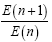in infinity is satisfied.

So from this we can write a recurrent relationship for E(n):

E(n+1)=A(n0-1)*E(n)+A(n0-2)*E(n-1)+A(n0-3)*E(n-2)+……… .A(1)*E(n-n0+2)+A(0)*E(n-n0+1)

Let’s define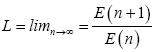E(n+1)/E(n)=A(n0-1)+A(n0-2)×[E(n-1)/E(n)]+A(n0-3)×[E(n- 2)/E(n)]+…

A(1)×[E(n-n0+2)/E(n)]+A(0)×[E(n-n0+1)/E(n)]

Let’s define G(n+1)=E(n+1)/E(n) so we can write:

G(n+1)=A(n0-1)+A(n0-2)/Gn+A(n0-3)/[Gn*G(n-1)]+………….+A(1)/[G(n)*G(n-1)*….G(n-n0+3)]+A(0)/[Gn*G(n-1)*…… G(n-n0+2)]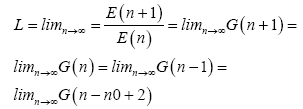So L=A(n0-1)+A(n0-2)/L+A(n0-3)/L2+……A(1)/ L(n0-2)+A(0)/L(n0-1)

Multiplication of the two sides by L(n0-1) leads to:

L(n0)=A(n0-1)*L(n0-1)+A(n0-2)*L(n0-2)+A(n0-3)*L(n0-3)+……. A(1)*L+A(0)

So X=L is a solution of the n0th polynomial equation

X(n0)=A(n0-1)*X (n0-1)+A(n0-2)*X (n0-2)+A(n0-3)*X (n0-3)…...A(1)*X+A(0).

Particular cases:

* When A(n0-1)=0

Before being able to solve the equation, we first need to transform the equation into a classical n0 order equation when at least the coefficient of xn0-1 and x0 will be different from 0. This can be done by setting X=x+a , then we can solve the new equation in x.

Example: Let’s get a look at the equation X3=3X+4

Substitution of X by x+a where “ a” can be any real leads to:

(x+a)3=3(x+a)+4

so developing the cubic form leads to:

x3+a3+3 a2x+3a x2=3x+3a+4 this leads to:

x3=-3a x2+x(3-3 a2)+(3a+4) – a3

So X=x+a is the solution of the cubic equation X3=3X+4

* If for a particular Ai≠0 A(n0-1)*A(i)+A(i-1)=0

We need to transform the equation for i by setting X=x+a and then we need to recalculate the next terms of the series E(k).

#### Complex Solutions

For a polynomial P(X)=0,P(a+bi)=P(abi)=0 so we need to solve separately for the real parts and imaginary parts so it sends to solve a regular polynomial equation at one variable to find a and b

Example

Let’s try to solve a seventic (n0=7) equation

X7=2X6+4X5+3X4+2X3+X2+X+6

Let’s express the recurrent relationship for E(n) and G(n):

E(n+1)=2E(n)+4E(n-1)+3E(n-2)+2E(n-3)+E(n-4)+E(n- 5)+6E(n-6)

G(n+1)=E(n+1)/E(n)

G(n+1)=2+4/G(n)+3/[G(n)*G(n-1)]+2/[G(n)*G(n-1)*G(n-2)]+1/[G(n)*G(n-1)*G(n-2)*G(n-3)]+1/[G(n)*G(n-1)*G(n-2)*G(n- 3)*G(n-4)]+6/[G(n)*G(n-1)*G(n-2)*G(n-3)*G(n-4)*G(n-5)]

Before we can use those formulas we need to calculate the first six terms for E(n) and

G(n):

E(8),E(9),E(10),E(11),E(12),E(13)

G(8), G(9), G(10), G(11), G(12), G(13)

X8=X7X=2[2X6+4X5+3X4+2X3+X2+X+6]+4X6+3X5+2X4+X3+X2+6X=8X6+11X5+8X4+5X3+3X2+8X+12

So E(8)=8 , from the seventic equation E(7)=2

So G(8)=E(8)/E(7)=8/2=4

X9=X8X=8X7+11X6+8X5+5X4+3X3+8X2+12X=

Substitution of X7 by the equation leads to:

X9=8[2X6+4X5+3X4+2X3+X2+X+6]+

11X6+8X5+5X4+3X3+8X2+12X=

27X6+40X5+29X4+19X3+16X2+20X+48

G(9)=E(9)/E(8)=27/8=3.375

X10=X9 *X=27[2X6+4X5+3X4+2X3+X2+X+6]+

40X6+29X5+19X4+16X3+20X2+48X=

94X6+137X5+100X4+70X3+47X2+75X+162

G(10)=E(10)/E(9)=94/27=3.481481481

X11=X10 *X = 94 [ 2 X6+4X5+3X4+2X3+X2+ X + 6 ] + 137X6+100X5+70X4+47X3+75X2+ 162X=325X6+476X5+352X4+235X3+169X2+256X+564

G(11)=E(11)/E(10)=325/94=3.457446809

X12=X11*X=325[2*X6+4X5+3X4+2X3+X2+X+6]+

476 X6+352 X5+235X4+169X3+256X2+564X=

1126X6+1652X5+1210X4+819X3+581X2+889 X+1950

G(12)=E(12)/E(11)=1126/325=3.464615385

X13=X12*X=1126[2X6+4X5+3X4+2X3+X2+X+6]+

1652X6+1210X5+819X4+581X3+889X2+1950X=3904X6+5714X5+4 197X4+2833X3+2015X2+3076X+6756

G(13)=E(13)/E(12)=3904/1126=3.46714032

So we are ready now to use the recurrent formula for G(n)

G(14)=2+4/G(13)+3/[G(13)*G(12)]+2/[G(13)*G(12)*G(11)]+

1/[G(13)*G(12)*G(11)*G(10)]+1/[G(13)*G(12)*G(11)* G(10)* G(9)]+

6/[G(13)*G(12)*G(11)*G(10)*G(9)*G(8)]=3.463627049

Applying the formula for the next terms of G(n) for n15 leads to:

G(15)=3.465241828

G(16)=3.46714032

G(17)=3.464868461

G(18)=3.464830021

G(19)=3.464840265

G(20)=3.464839802

G(21)=3.464838453

G(22)=3.464839319

G(23)=3.46483897

G(24)=3.464839088

G(25)=3.46483905

G(26)=3.464839061

G(27)=3.464839059

G(28)=3.464839058

G(29)=3.464839059

G(30)=3.464839059

Since G(29)=G(30)=3.464839059

So we can conclude that all the next terms of the series for n≥30 will be equal to G(30)

So we can conclude that L= limn→∞G (n) =3.464839059 checking this result will show that

X=L=3.464839059 is a solution of the seventic equation

In this example, we saw that in fact (considering a finite number of digits after the decimal point) a finite number of iterations is needed to calculate the limit L.

So let’s formulate a general result for the number of iterations needed to calculate the limit L.

Let it be a no th polynomial equation:

Xn0=A(n0-1)*Xn0-1+A(n0-2)*Xn0-2+A(n0-3)*Xn0-3+….A(1)*X+A(0)

Let it be the coefficients[A(n0-1), A(n0-2), A(n0-3),A(n0-4),… ..A(0)] real integers different from zero [A(i) integers A(i)≠0 and A(i)∈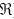]

The number of iterations needed is determined by the minimum common factor Ko.

Ko is the minimum common factor between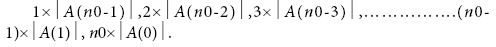Ko is determined by simplifying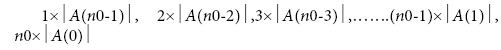by each pair of prime factors (p,p) contained in the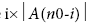for i=1,2,3,…….(n0-1) and then by taking the product between the different prime factors in the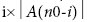,

Then we determine the equivalent of the product in modulo n0 the result is k0[n0],

So ko+n0 iterations will always give at least one digit of the root solution X.

Then for m digits the number of iterations that will always give the m digits is

K=( Ko+n0)×[1+2+…..m]=( Ko+n0)×[m*(m+1)/2].

In the decimal system K+10 iterations will be the maximum number of iterations needed that the m digits are also common to the subsequent terms of the serie G(n).

This says that for k≥ K+10

k iterations correspond to the indice k+n0 for the serie G(n)

So G(k+n0) contains the m digits of the root solution.

So X(with m digits)=

Int[G[(Ko+n0)*m*(m+1)/2+n0+10] ×10(m)]/ 10(m)

If we call k1=Ko+n0 and Int the integer value of a parameter we can express a general formula for the solution of polynomial equations:

X(with m digits)=Int[G[k1*m*(m+1)/2+10]×10(m)]/10(m)

For calculating Ko, a rule is that only the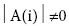are taken into account for the product. In any case the minimum condition is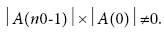A particular case:

When [A(n0-1), A(n0-2), A(n0-3),………..A(0)] are not integers we express their rational form A(i)=A’(i)/A’(n0)

So we can express the equation in this form

A’(n0)* X (n)=A’(n0-1)*X (n0-1)+A’(n0-2)*X(n0-2)+…..A’(1)*X+A’(0)

[A’(n0), A’(n0-1), A’(n0-2),……….A’(0)] ≠0 and also real integers.

Then we can express the product for Ko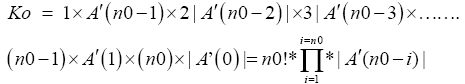A rule is that only the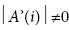are taken into account for the product. In any case the minimum condition is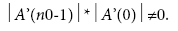Now the question which can be raised up is how to know the number of digits which a rational solution may have.

Determination of the maximum number of digits for a rational solution in the decimal base

Let it be a n0 th polynomial equation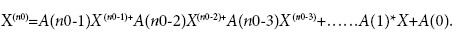If X has m digits in the decimal base so the number of digits of the left side can be expressed Am+B and in the right side can be expressed Cm+D,So since the left side is equal to the right side so Am+B=Cm+D

(A-C)*m=D-B so m=(D-B)/(A-C) The biggest value for m can be obtained for D maximum, B minimum, A minimum, C maximum C maximum=n0-1A minimum=n0B minimum=-n0+1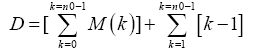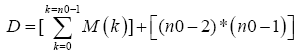So m=[D+(n0-1)]/[n0-(n0-1)]=D+n0-1

So m ≤ D+n0-1

So m(Max)=D+n0-1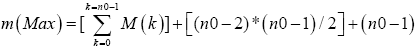When M(k) is the number of digits for A(k): example if A(n0- 1)=13 so M(n0-1)=2.

What Henrik Niels Abel has demonstrated is that a general formula for the solution of polynomial equations can not be obtained in terms of radicals for degree n ≥ 5 .

However the equation X2=2 is in itself a mathematical problem to solve because saying that the solution of this equation is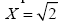does not give the arithmetic expression of the solution

In order to solve the equation X2=2 we need first to transform this equation into a classical second order equation. This can be done by transformation with X=x+a

(x+a)2=2 this leads to:

x2+a2+2ax=2⇔ x2=-2ax+2-a2.

One example that will lead to the solution can be a=1 this leads to x2=-2x+2-1=-2x+1

so we need to solve the equation.

x2=-2x+1 then X=x+1 corresponds to the solution of X2=2 or in other terms we can express the square root of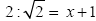.

Let’s solve the equation

x2=-2x+1

Let’s express the recurrent relationship for E(n) and G(n)

E(n+1)=-2E(n)+E(n-1)

G(n+1)=-2+[1/G(n)]

Before we can use those formulas we need first to calculate the first terms for E(n) and

G(n): E(3),G (3)

x3=x2*x=-2x2+x=-2[-2x+1]+x=4x –2+x=5x-2

So E(3)=5, G(3)=E(3)/E(2)=5/(-2)=-2.5

Applying the formula for G(n) leads to:

G(4)=-2+[1/(-5/2)]=-2+(-2/5)=-2-0.4=-2.4

G(4)=-2.4

G(5)=-2.416666667

G(6)=-2.413793103

G(7)=-2.414285714

G(8)=-2.414201183

G(9)=-2.414215686

G(10)=-2.414213198

G(11)=-2.414213625

G(12)=-2.414213552

G(13)=-2.414213564

G(14)=-2.414213562

G(15)=-2.414213562

Since G(15)=G(14)=-2.414213562.

So we can conclude that x1=-2.414213562 we can now find easily the other solution

1 2-1(-2.414213562)

1 (-2.414213562+2) 0

The other solution is x2=2.414213562-2=0.414213562

X1=x+1=-2.414213562+1=-1.414213562

X2=0.414213562+1=1.414213562

So those are the two square roots of 2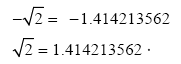So my conclusion is that since we can find the radicals in terms of simple operations like additions multiplications subtractions divisions so we should be able to find general formulas which can be expressed in terms of simple operations to express the solutions of any nth polynomial equation. All what is needed for this is to find explicit formulas for the series E(n) and G(n).

Let’s try to develop a formula for the second degree polynomial equations. X2=PX+Q

let’s express the recurrent relationship for E(n):

E(n+1)=P*E(n)+Q*E(n-1)

This is the formula for E(k):when k=0 corresponds to n=n0=2 (k=n-n0=n-2)

E(k)=P (k+1)+k [P(k-1)]×Q+[(k-2)(k-1)/2]× [P(k-3)]×Q2+

[ (k-2)(k-3)(k-4)/6]×[P (k-5)]×Q3+[(k-3)(k-4)(k-5)(k-6)/24]×[P(k-7)]×Q4

+[(k-4)(k-5)(k-6)(k-7)(k-8)/120]×[P(k-9)]×Q5+……………..

The final development of the formula leads to: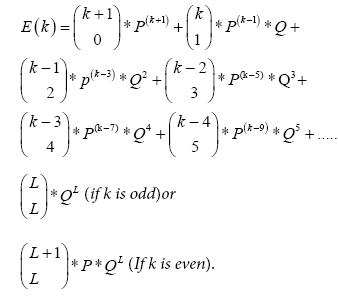The last term of the formula will depend on whether k is odd or k is even

Now we will try to obtain a general formula valid for any n0 th degree polynomial equation with coefficients A(n0-1),A(n0-2), A(n0- 3),……..A(1),A(0) :

X(n0)=A(n0-1)*X(n0-1)+A(n0-2)*X(n0-2)+A(n0-3)*X(n0-3)+…… .A(1)*X+A(0)

Let’s express the recurrent relationship for E(k):

E(k+1)=A(n0-1)*E(k)+A(n0-2)*E(k-1)+A(n0-3)*E(k-2)+……… A(1)*E(k-(n0-2))+A(0)*E(k-(n0-1))

Developing a formula for E(k) leads to: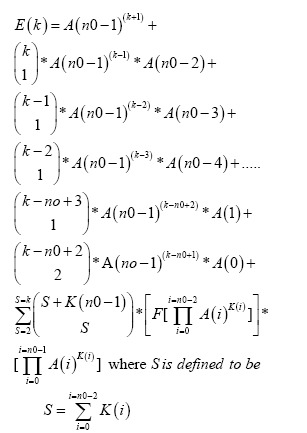K(n0-1) is defined to be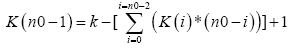with one condition: K(n0-1)≥ 0

and F is a function defined as this: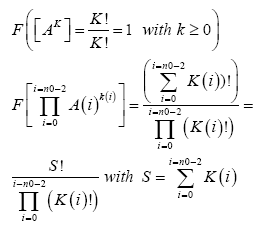In the formula for the serie E(k)

Example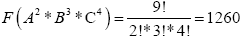We can rewrite the formula for E(k):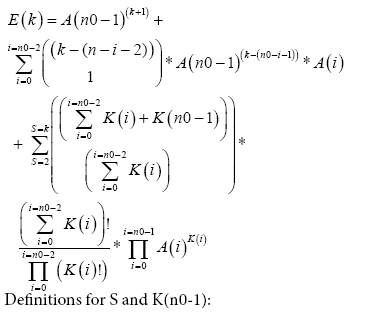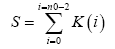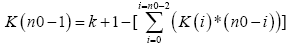Condition K(n0-1)≥ 0

This formula can be rewritten in the following expression: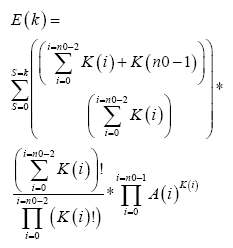Definitions for S and K(n0-1):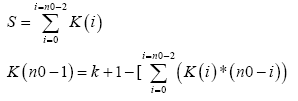condition K(n0-1)≥ 0

Now we will develop the formula E(k) for the quintic equation

X5=aX4+bX3+cX2+dX+e

The expression of Ek developed for the qunitic formula.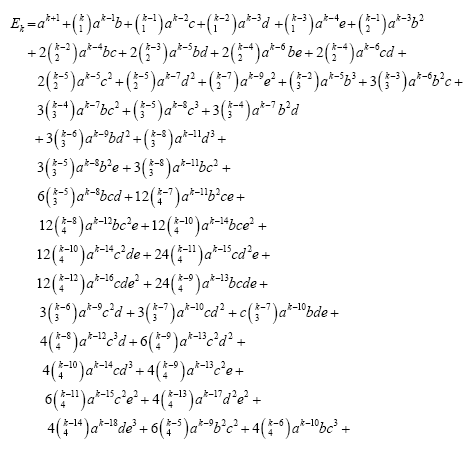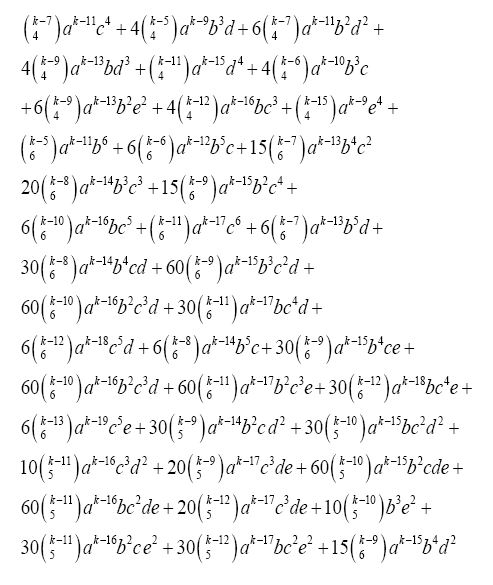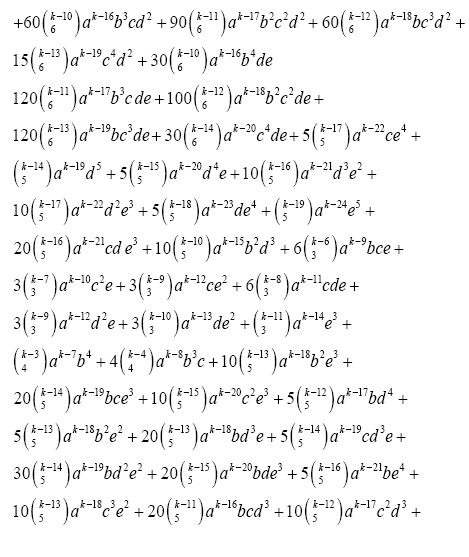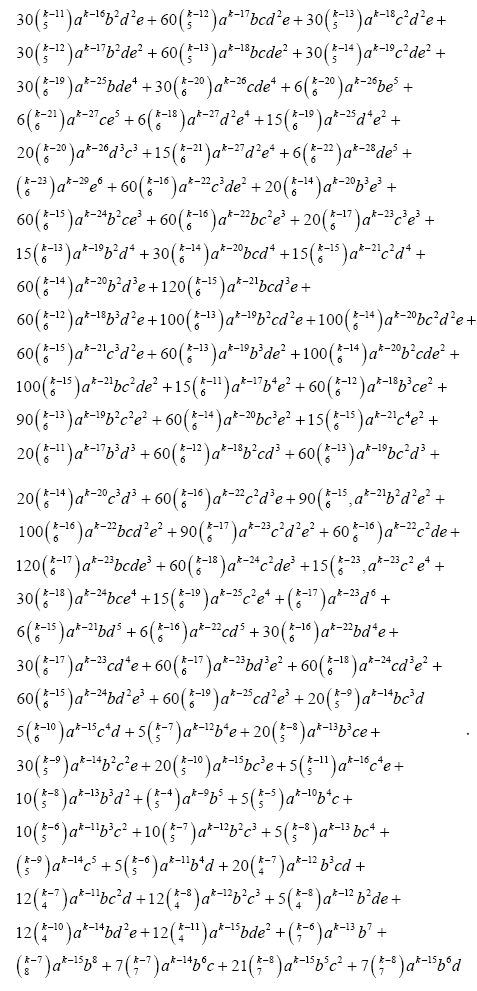Let’s express the root of a quantic equation by using the expression of Ek developed for the quinitic formula (n0=5)

X5=3X4+4X3+2X2+X+1.

The first thing we need to determine is the minimum number of interaction needed .to calculate the solution of the equation. This number is called K1=K0+n0 following the derivatives given to determine Kc.

We illustrate how to calculate K0 , K0 is the minimum common factor between

1×3 ,2×4,3×2,4×1 ,5×1.

Where on the right side of each product is represented the coefficient of the quinitic equation and on the left side of each product is represented the indices starting from 1.

So we need to identify the different pair of prime factors contained in the product between the five products.

[1×3]×[2×4]×[3×2]×[4×1]×[5×1].

At next we need to express this product in terms of prime factors

[1×3]×[2×2×2]×[3×2]×[2×2×1]×[ 5×1].

The next thing we need to do is to divide this product by each pair of prime factors Let’s do this for each pair of prime factors

[1×3]×[2×2×2]×[3×2]×[2×2×1]×[5×1]/2×2×2×2×2×3×3=5.

At next we need to determine the equivalent of this result in terms of modulo n0 when n0 is the degree of the equation since the equation is a quintic equation so n0=5.

So 5 modulo 5=5=0

So k0=0 and k1=k0+n0=0+5=5

So K1=5

So K1=5 interacts will give at least one digit after the decimal point of the equation.

For m digits after the decimal point So:

K = (Ko + n0)[1+ 2…..m] = (Ko + n0) *m(m +1) / 2

This number k is the maximum number of the interactions needed to calculate the solutions until m digits after the decimal point.

So here k = 5m*(m +1) / 2

For m =1 K = 5*1*(1+1) / 2 = 5

In the decimal system K+10=5+10=15 interactions is the maximum number of the interactions for which m=1 digits after the decimal point is also common to the subsequent terms of the series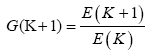which means that

L(with m=1 digit)=G(15)=G(16)

Infact checking the result with a calculator will show that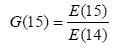gives 9 digits after the decimal point of the solution x.

So X (with 9 digits after the decimal point)=[int(G(15)*109)]/109

Where int is the integers function Now we need to calculate E(15) and E(14) using the expression of E(k) developed for the quantic formula we need to remember that only positive powers are allowed in the expression.

We calculate E(14) So k=14 ,a=3,b=4,c=2,d=1,e=1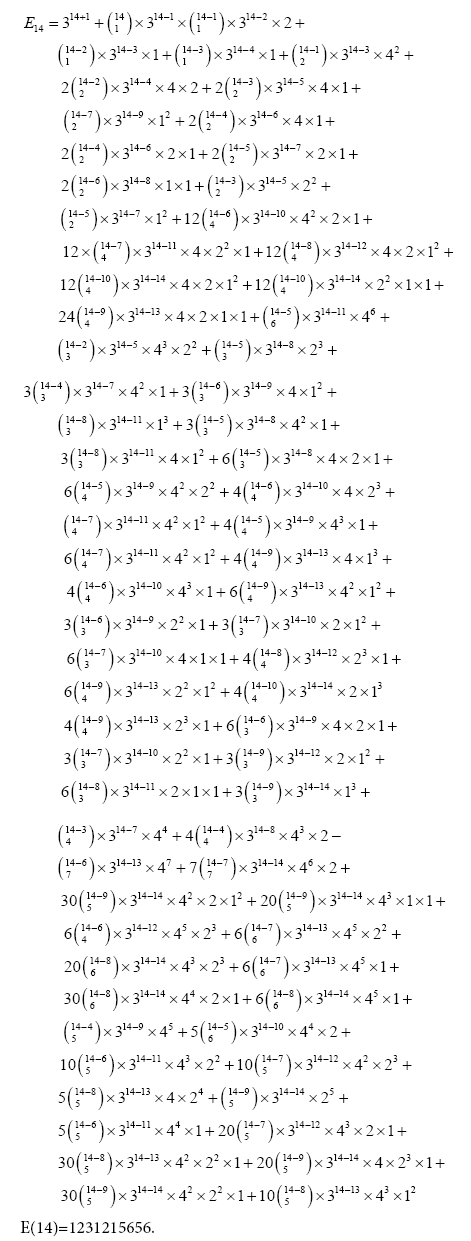At next we calculate E15 , k=15 a=3,b=4,c=2,d=1,e=1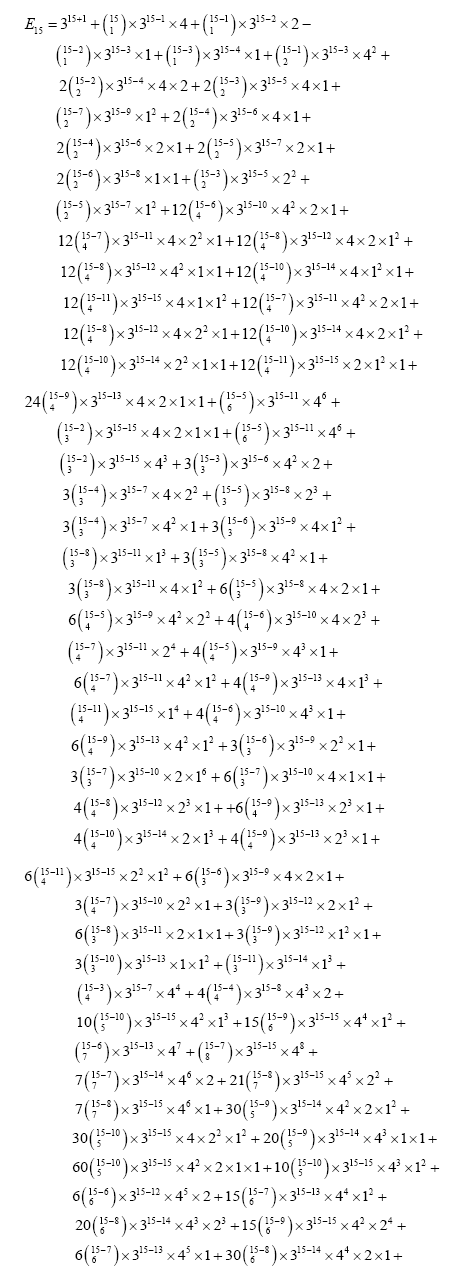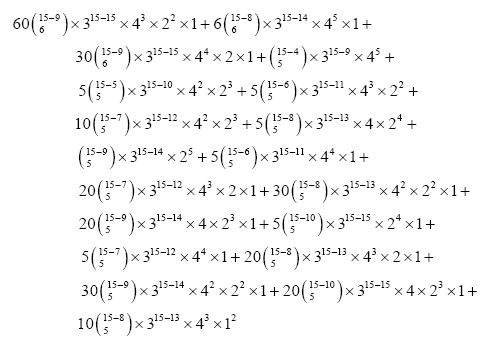E(15)=5059866125.

So let’s calculate the solution of the quantic equation: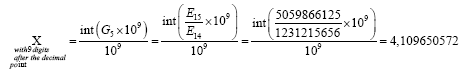So X=4.109650572 is a solution of the quantic equation. X5=3X4+4X3+2X2+X+1.

This result can be checked by the Newton method or any other technique.

This How J determined the different power combinations in the E(K) formula for K=15 and K=14.

Let’s denote K0,K1,K2,K3 the respective process of b,c,d,e in the qunitic equation.

X5=aX4+bX3+cX2+dX+e

For k0+k1+k2+k3=0 there is only one possibility k0=0, k1=0, k2=0, k3=0.

For k0+k1+k2+k3=1 this is equivalent to (k0+k1)+(k2+k3)=1 , so the possibilities for this are

(k0+k1)=0, (k2+k3)=1 or (k0+k1)=1, (k2+k3)=0

For (k0+k1)=1 the possibilities are k0=0 , k1=0 .

For (k2+k3)=1 the possibilities are k2=0 , k3=1 or k2=1 , k3=0.

For (k0+k1)=1 the possibilities are k2=0 , k1=1 or k0=1 , k1=0

For (k2+k3)=0 the possibilities are k2=0 , k3=0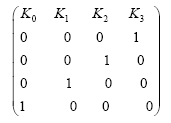This gives the followings different power combinations for bk0 ck1 dk2 ek3

b0c0d0e=e

b0c0de0=d

b0cd0e0=c

bc0d0e0=b

So we need to perform the same things

For K0+K1+K2+K3=2, K0+K1+K2+K3=3

K0+K1+K2+K3=4 , K0+K1+K2+K3=5 , K0+K1+K2+K3=6, K0+K1+K2+K3=7.

For the qunitic equation X5=3x4+4x3+2x2+x+1.

We needed E(15) and E(14)

For k0+k1+k2+k3>7 the powers of a=3 in E(15) will be negative and since the condition in the E(k)formula is K(no-1)≥ 0 , which means that the powers of a is to be positive powers .So we need to step the summation k0+k1+k2+k3 at the sum equal to 7 to calculate E(15).

These are the tables for the sum k0+k1+k2+k3 (Table 1).

Table 1: The summation k0+k1+k2+k3 at the sum equal to 7.

 K0+ k1+ k2+ k3=2 0 0 0 2 0 0 1 1 0 0 2 0 0 1 0 1 0 1 1 0 1 0 0 1 1 0 1 0 0 2 0 0 1 1 0 0 2 0 0 0 K0+ k1+ k2+ k3=3 0 0 0 3 0 0 1 2 0 0 2 1 0 0 3 0 0 1 0 2 1 0 0 2 0 1 1 1 1 0 1 1 0 1 2 0 1 0 2 0 0 2 0 1 1 1 0 1 2 0 0 1 0 2 1 0 1 1 1 0 2 0 1 0 3 0 0 0 2 1 0 0 1 2 0 0 0 3 0 0 K0+ k1+ k2+ k3=4 2 0 2 0 0 3 0 1 1 2 0 1 2 1 0 1 3 0 0 1 0 3 1 0 1 2 1 0 2 1 1 0 3 0 1 0 0 4 0 0 1 3 0 0 2 2 0 0 3 1 0 0 4 0 0 0 K0+ k1+ k2+ k3=5 0 0 0 5 0 0 1 4 0 0 2 3 0 0 3 2 0 0 4 1 0 0 5 0 0 1 0 4 1 0 0 4 0 1 1 3 1 0 1 3 0 1 2 2 1 0 2 2 0 1 3 1 1 0 3 1 0 1 4 0 1 0 4 0 0 2 0 3 1 1 0 3 2 0 0 3 0 2 1 2 1 1 1 2 K0+ k1+ k2+ k3=5 2 0 1 2 0 2 2 1 1 1 2 1 2 0 2 1 0 2 3 0 1 1 3 0 2 0 3 0 0 3 0 2 1 2 0 2 2 1 0 2 3 0 0 2 0 3 1 1 1 2 1 1 2 1 1 1 3 0 1 1 0 3 2 0 1 2 2 0 2 1 2 0 3 0 2 0 0 4 0 1 1 3 0 1 K0+ k1+ k2+ k3=5 2 2 0 1 3 1 0 1 4 0 0 1 0 4 1 0 1 3 1 0 2 2 1 0 3 1 1 0 4 0 1 0 0 5 0 0 1 4 0 0 2 3 0 0 3 2 0 0 4 1 0 0 5 0 0 0 K0 + k1+ k2+ k3=6 0 0 0 6 0 0 1 5 0 0 2 4 0 0 3 3 0 0 4 2 0 0 5 1 0 0 6 0 0 1 0 5 1 0 0 5 0 1 1 4 1 0 1 4 0 1 2 3 1 0 2 3 0 1 3 2 1 0 3 2 0 1 4 1 1 0 4 1 0 1 5 0 1 0 5 0 0 2 0 4 K0+ k1+ k2+ k3=6 1 1 0 4 2 0 0 4 0 2 1 3 1 1 1 3 2 0 1 3 0 2 2 2 1 1 2 2 2 0 2 2 0 2 3 1 1 1 3 1 2 0 3 1 0 2 4 0 1 1 4 0 2 0 4 0 0 3 0 3 1 2 0 3 2 1 0 3 3 0 0 3 0 3 1 2 1 2 1 2 2 1 1 2 K0   + k1   + k2   + k3=6 3 0 1 2 0 3 2 1 1 2 2 1 2 1 2 1 3 0 2 1 0 3 3 0 1 2 3 0 2 1 3 0 3 0 3 0 0 4 0 2 1 3 0 2 2 2 0 2 3 1 0 2 4 0 0 1 0 4 1 1 1 3 1 1 2 2 1 1 3 1 1 1 4 0 1 1 0 4 2 0 1 3 2 0 K0   + k1  + k2+ k3=6 2 2 2 0 3 1 2 0 4 0 2 0 0 5 0 1 1 4 0 1 2 3 0 1 3 2 0 1 4 1 0 1 5 0 0 1 0 5 1 0 1 4 1 0 2 3 1 0 3 2 1 0 4 1 1 0 5 0 1 0 0 6 0 0 1 5 0 0 2 4 0 0 3 3 0 0 4 2 0 0 5 1 0 0 K0+ k1+ k2+ k3=6 6 0 0 0 K0+ k1+ k2+ k3=7 0 0 0 7 0 0 1 6 0 0 2 5 0 0 3 4 0 0 4 3 0 0 5 2 0 0 6 1 0 0 7 0 0 1 0 6 1 0 0 6 0 1 1 5 1 0 1 5 0 1 2 4 1 0 2 4 0 1 3 3 1 0 3 3 0 1 4 2 1 0 4 2 0 1 5 1 K0+ k1+ k2+ k3=7 1 0 5 1 0 1 6 0 1 0 6 0 0 2 0 5 1 1 0 5 2 0 0 5 0 2 1 4 1 1 1 4 2 0 1 4 0 2 2 3 1 1 2 3 2 0 2 3 0 2 3 2 1 1 3 2 2 0 3 2 0 2 4 1 1 1 4 1 2 0 4 1 0 2 5 0 1 1 5 0 2 0 5 0 K0+ k1 k2 k3=7 0 3 0 4 1 2 0 4 2 1 0 4 3 0 0 4 0 3 1 3 1 2 1 3 2 1 1 3 3 0 1 3 0 3 2 2 1 2 2 2 2 1 2 2 3 0 2 2 0 3 3 1 1 2 3 1 2 1 3 1 3 0 3 1 0 3 4 0 1 2 4 0 2 1 4 0 3 0 4 0 0 4 0 3 K0 + k1+ k2+ k3=7 1 3 0 3 2 2 0 3 3 1 0 3 4 0 0 3 0 4 1 2 1 3 1 2 2 2 1 2 3 1 1 2 4 0 1 2 0 4 2 1 1 3 2 1 2 2 2 1 3 1 2 1 4 0 2 1 0 4 3 0 1 3 3 0 2 2 3 0 3 1 3 0 4 0 3 0 0 5 0 2 1 4 0 2 K0+ k1+ k2+ k3=7 2 3 0 2 3 2 0 2 4 1 0 2 5 0 0 2 0 5 1 1 1 4 1 1 2 3 1 1 3 2 1 1 4 1 1 1 5 0 1 1 0 5 2 0 1 4 2 0 2 3 2 0 3 2 2 0 4 1 2 0 5 0 2 0 0 6 0 1 1 5 0 1 2 4 0 1 3 3 0 1 4 2 0 1 K0    + k1    + k2    + k3=7 5 1 0 1 6 0 0 1 0 6 1 0 1 5 1 0 2 4 1 0 3 3 1 0 4 2 1 0 5 1 1 0 6 0 1 0 0 7 0 0 1 6 0 0 2 5 0 0 3 4 0 0 4 3 0 0 5 2 0 0 6 1 0 0 7 0 0 0

Table 1: The summation k0+k1+k2+k3 at the sum equal to 7.

#### References

Citation: Julien Y (2018) Method for Solving Polynomial Equations. J Appl Computat Math 7: 409. DOI: 10.4172/2168-9679.1000409

Copyright: © 2018 Julien Y. This is an open-access article distributed under the terms of the Creative Commons Attribution License, which permits unrestricted use, distribution, and reproduction in any medium, provided the original author and source are credited.

Select your language of interest to view the total content in your interested language

##### Recommended Journals
Viewmore
###### Article Usage
• Total views: 938
• [From(publication date): 0-2018 - Aug 26, 2019]
• Breakdown by view type
• HTML page views: 888
• PDF downloads: 50

## Post your commentCan't read the image? click here to refresh
###### Peer Reviewed Journals

Make the best use of Scientific Research and information from our 700 + peer reviewed, Open Access Journals

International Conferences 2019-20

Meet Inspiring Speakers and Experts at our 3000+ Global Annual Meetings

Top